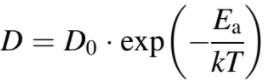Electromigration
- Practical Electron Microscopy and Database -
- An Online Book -

http://www.globalsino.com/EM/

=================================================================================

The mechanism of the interaction between the current carriers and mobile atoms in materials is not fully understood, but it is generally accepted that electrons moving in conductors are continuously scattered by defects. Electromigration (EM) is a term applied to the transport of mass in both molten and solid metals when the materials are stressed at high current densities, since the sufficient electron momentum is imparted to atoms to physically propel them into activated configurations and then toward the anode. Therefore, the atomic motion is not determined only by the electrostatic (Coulomb) force imposed by the applied field but also by the direction of motion of the charge carriers.

In other words, electromigration is the transport of atoms induced by the gradual displacement of ions in a conductor or semiconductor due to the momentum transfer between conducting electrons and diffusing metal atoms. This effect occurs when the current density is high enough to cause the drift of ions in the direction of the electron flow, and is characterized by the ion flux density. The electromigration mechanisms are related to the nature of the conductor, crystal size, interface and grain-boundary chemistry, and the magnitude of forces such as the current density, temperature and mechanical stresses. As the structure size in electronics such as integrated circuits (ICs) decreases, the practical significance of the electromigration effect increases.

The driving forces for electromigration can be categorized by the two components,
i) purely electrostatic force. In this case, the accelerated electrons "shield" the ion to reduce the electric field influence.
ii) interaction with moving charge carriers, e.g. electron wind force. This electron "wind" force is oppositely directed to and is normally larger than the well-shielded electrostatic force on atom cores arising from the applied electric field.

The total driving force on a moving ion in the EM process consists of two components and can be given by,
F = Fwind + Fstatic ---------------------- [2893a]
where,
Fwind -- the electron wind force,
Fstatic -- the electrostatic force.

The electron wind force Fwind is normally larger than the electrostatic force Fstatic, and thus the momentum exchange between the electron wind and ions (or atoms) is normally the dominant mechanism for electromigration.

In theoretical treatment, the driving force is usually given in terms of an effective charge Z*,
Feff = |e|Z*ξ ---------------------- [2893b]
where,
|e| -- the absolute value of the electronic charge.
ξ -- the electric field (= -ϕ).
Z* -- the effective charge of the solute (=nλσ-Z).
Z -- the charge of the ion.
λ -- the mean free path.
σ -- the atom's intrinsic cross section for collision with the electrons.

The sign of Z* can be positive or negative, depending on the properties of the materials, for instance, a positive electric field gives a negative force.

The atomic flux (Ja) in the direction of the electron wind under the EM condition can be given by
Ja = µ·Feff -------------------------- [2893c]
where,
C -- the concentration (or so-called atomic density) of the solute (e.g. ions),

Substituting the electromigration driving force from Equation 2893b into Equation 2893c, the flux of a solute can then be given by,
Ja = C·µ·|e|·Z*·ξ = C·µ·|e|·Z*·ρ·J -------------------------- [2893d]
where,
µ -- the mobility of the solute.
ρ -- the resistivity of the materials.
J -- the current density.

According to the Einstein relation, the atomic mobility can be given in terms of the diffusion coefficient by,
µ = D/kT -------------------------- [2893e]
where,
D -- the diffusion coefficient for the solute (e.g. metal atoms),
k -- Boltzmann’s constant,
T -- the absolute temperature.

On the other hand, the DZ* value represents the atomic mobility in electromigration, which do not depend on the external conditions, such as electric field, initial concentration, and temperature. Table 2893a gives the effective charge numbers of some elements in electromigration.

Table 2893a. Effective charge numbers (Z*) of some elements in electromigration.

 Element Z* Reference Ge 0.63  Sb 0.57  Te -0.45 

The diffusion coefficient of the solute can be represented by,-------------------------- [2893f]
where,
Ea -- the activation energy.
T -- the diffusion coefficient at room temperature.

Mechanisms in electromigration process can be categorized by:
i) The metallurgical-statistical properties of the materials. The metallurgical statistical properties of the materials dependes on the microstructure of the materials, including amorphous properties, grain size, grain boundary, grain orientation and their distributions with respect to electron flow.
ii) The thermal accelerating process due to the local increase in temperature. Before any electromigration damage occurs, the temperature distribution in the materials is uniform. With the formation of a void, the temperature of the area, in which the current flow is reduced, decreases, while the current density increases in the area where the current crowds. Those phenomena changes the temperature distribution in the material.
iii) The healing effects. Electrical static force, in the direction opposite to the electron wind force, during or after electromigration is called back-flow, which causes the healing effect. This back-flow of mass can be due to inhomogenities of temperature and/or concentration gradients caused by the electromigration process.

Table 2893b. Electromigration (EM) failure in very large scale integrated (VLSI) circuits.

Material
Failure probability
Al Much more EM-induced failures than Au
Au Much less EM-induced failures than Al
Grain boundary Very large

 Tae-Youl Yang, Ju-Young Cho, Yong-Jin Park, and Young-Chang Joo, Effects of dopings on the electric-field-induced atomic migration and void formation in Ge2Sb2Te5, 18th IEEE International Symposium on the Physical and Failure Analysis of Integrated Circuits (IPFA), DOI: 10.1109/IPFA.2011.5992717, (2011).

=================================================================================